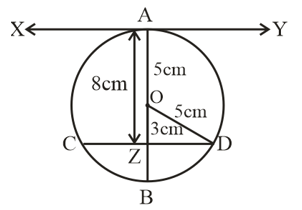#### At one end A of a diameter AB of a circle of radius 5 cm, tangent XAY is drawn to the circle. The length of the chord CD parallel to XY and at a distance 8 cm from A is(A) 4 cm                      (B) 5 cm                                  (C) 6 cm                      (D) 8 cmSolution
According to questionsGiven AO = OB = 5 cm
Distance between XY and CD = 8 cm
Since D is the center of the circle and CD is chord. If we join OD it become the radius of circle that is OD = 5cm
AZ = 8 cm (given)
AZ = AO + OZ
8 = 5 + OZ
OZ = 3cm
In ODZ, use Pythagoras theorem

Length of chord CD = 8 cm